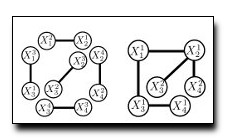Covering Trees and Lower-bounds on Quadratic AssignmentMany computer vision problems involving feature correspondence among images can be formulated as an assignment problem with a quadratic cost function. Such problems are computationally infeasible in general but recent advances in discrete optimization such as tree-reweighted belief propagation (TRW) often provide high-quality solutions. In this paper, we improve upon these algorithms in two ways. First, we introduce covering trees, a variant of TRW which provide the same bounds on the MAP energy as TRW with far fewer variational parameters. Optimization of these parameters can be carried out efficiently using either fixed-point iterations (as in TRW) or sub-gradient based techniques. Second, we introduce a new technique that utilizes bipartite matching applied to the min-marginals produced with covering trees in order to compute a tighter lower-bound for the quadratic assignment problem. We apply this machinery to the problem of finding correspondences with pairwise energy functions, and demonstrate the resulting hybrid method outperforms TRW alone and a recent related subproblem decomposition algorithm on benchmark image correspondence problems.

### Text Reference

Julian Yarkony, Charless Fowlkes, and Alex Ihler. Covering trees and lower-bounds on quadratic assignment. In CVPR. 2010.

### BibTeX Reference

@inproceedings{YarkonyFI_CVPR_2010,
AUTHOR = "Yarkony, Julian and Fowlkes, Charless and Ihler, Alex",
TITLE = "Covering Trees and Lower-bounds on Quadratic Assignment",
BOOKTITLE = "CVPR",
YEAR = "2010",
TAG = "grouping"
}